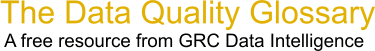Choose Index below for a list of all words and phrases defined in this glossary.

# A-Square

index | Index

## A-Square - definition(s)

A-Square - A-squared is the test statistic for the Anderson-Darling Normality test. It is a measure of how closely a dataset follows the normal distribution. The null hypothesis for this test is that the data is normal. So if you get an A-squared that is fairly large, then you will get a small p-value and thus reject the null hypothesis. Small A-squared values imply large p-values, thus you cannot reject the null hypothesis.

[Category=Data Quality ]

Source: iSixSigma, 29 December 2010 09:02:28, http://www.isixsigma.com/index.php?option=com_glossaryData Quality Glossary.  A free resource from GRC Data Intelligence. For comments, questions or feedback: dqglossary@grcdi.nl